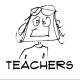# Fractions are Everywhere! (Lesson Plan)

This lesson was kinda fun. It shows students that you can find fractions everywhere… even in Hershey’s chocolate bars.  I wish I had been rich and could give each student their own chocolate bar to give the lesson extra punch, but alas, I am a teacher…

Context
Name: XXXXXX                         Date: March 12, 2009                         Grade level: 3rd
Subject/topic: Fractions                Length/minutes: 20-30 minutes          Group size: whole class
Sequence: ongoing
Purpose
Standard/core: UtahStandard 1, objective 2, indicator b
Standard 1
Students will understand the base-ten numeration system, place value concepts, simple fractions and perform operations with whole numbers.
Objective 2
Use fractions to communicate and compare parts of the whole.
a.   Identify the denominator of a fraction as the number of equal parts of the unit whole and the numerator of a fraction as the number of equal parts being considered.
b.  Define regions and sets of objects as a whole and divide the whole into equal parts using a variety of objects, models, and illustrations.
Learning goal: Students will understand that they can make fractions out of anything.
Major concepts:
Fraction- part or portion of a whole
Denominator- bottom number of a fraction; the number of pieces in the whole
Numerator- top number of a fraction; number of pieces we are looking at
Equivalent fractions- same size; equal fractions; fractions with the same portion of the whole shaded
Assessment
Given a worksheet, students will be able to draw a fraction (illustrated with objects of their choice).
Management
Self starter: none
Expectations: Sit and raise hands (no calling out); Students will focus on the topic; No sharpening pencils, getting out of seats going to the bathroom during the lesson.
Procedures: work with your table buddy; raise hands to speak.
Fast finisher: Color your picture if you finish early.
Instructional Strategies

Attitude Orientation: You probably already use fractions more than you realize. Name some ways you personally have used fractions…

Tell objective: “Today, are going to see how many things we can make fractions out of.”
Schema Orientation:
Using a Power Point Slide, show pages from The Hershey’s Fraction Book that illustrate common fractions. Use the following outline to help move through the Power Point. Italics indicate speech.
Slide 2: Picture of a Hershey’s candy bar. The candy bar is the whole.
Slide 3: How many pieces make the whole? Wait and let students count the pieces in the bar.
Slide 4:Point out each piece. 12 pieces make up the whole.
Slide 5: How do we write this as a fraction?
Slide 6: Since we just counted 12 pieces, that’s how big each piece is, 1/12 of the total. The total number of pieces goes at the bottom. Remember how we used the cubes yesterday during centers? The denominator told us which size piece we had. That’s our denominator, which goes on the bottom of the fraction. (Write it on the board). Now for the top of the fraction. How many do we have? We just counted 12, so that’s the numerator. (Write it on the board).
We can use this candy bar to make more than one fraction.
Slide 7: If we eat one piece, what fraction of the bar is left?
Slide 8: Let’s see. There are still 12 pieces total, so that’s our denominator. (Write it on the board). But our numerator has changed. Now how many are there? There are 11 left. So what’s the fraction left? 11/12.
Slide 9: Can we make fractions out of other things?
Slide 10: discuss how there are 5 cows on the page, and one of them is brown. 1/5 of the cows are brown.
Slide 11: Is there anything else that we can make into a fraction?
Slide 12: Cacao beans. Explain how 1/8 of the beans are falling out of the tree.
Slide 13: So, you can make fractions out of anything!
Activity:
Model how to use manipulative:
For example, if I found these leaves on the ground, I can make a fraction out of them. Put them on the board and show how to make a fraction. Model finding the total, and then the number that are different (example: 3 of the 9 leaves are orange). Give students the thought process behind finding a hidden fraction in a set of objects.
Since we just learned that we can make fractions out of anything, let’s try to make some.
(Pass out bags of objects- one per student).
Slide 13:Find the hidden fraction in your set of things.  Teacher may want to suggest ways to look at fractions (by color, by type of object, etc.)
Check for understanding:
As students make their hidden fractions, walk around the room and see if students are understanding the concept.
Independent practice:
Slide 14: Draw a picture of the fractions on your worksheet.
Pass out worksheet and ask students to draw objects of their choice to illustrate the fractions.
Closure: Lead a class discussion about the importance of fractions and the ways we see them in everyday life. So now that you know how to make anything a fraction, see how many fractions you can find around you every day!
Accommodations
Visual learners- Power Point visuals, charts, drawing assessment
ELL students- Modeling, pictures to match the words, hands-on activities
Resources
Power Point presentation
Projector
Computer
Manipulatives
Worksheet
Reflection
Step 1: (Instruction and Management) What went well? What should be improved?
Step 2: (Student Learning) What did the children learn? How do you know?
What have you found helpful when teaching fractions?

## 2 thoughts on “Fractions are Everywhere! (Lesson Plan)”

1. This is a great book for teaching kids fractions.

•squareheadteachers on said:

Agreed!

This site uses Akismet to reduce spam. Learn how your comment data is processed.